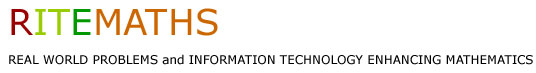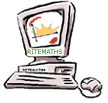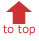Project Home Publications & Seminars Research Materials Project Team Only

Resources for using SMG (Symbolic Math Guide)

Symbolic Math Guide is a program written for the TI-89 calculator, which supports students who are learning to carry out algebraic manipulations.

Click here to access the SMG notes prepared by RITEMATHS team and guide to prepared files.

Resources

They provide a sample and a set of exercises on each topic. These exercises sets can easily be extended by teachers or students.

Linear equation files

These six files contain equations involving brackets and fractions, equations where the variable appears on both sides of the equation, equations where some work has to be done before the x's can be collected together and revision exercises.

LinEqFiles.zip

These six files contain equations involving the null factor law, the use of perfect squares, the use of factorisation, the use of the quadratic formula, rearrangement, (including simplification of rational fractions and /or cross multiplication), challenging exercises and revision exercises.

Calculus

Derivatives

These five files contain derivatives of polynomials, derivatives requiring use of the product, the quotient and the chain rule, and revision exercises.

Integrals

These six files contain indefinite integrals involving polynomials, exponential and trigonometric functions, indefinite integrals which may be evaluated by using the method of change of variable (use of substitution), including use of the double angle formula for trigonometric functions, indefinite integrals of logarithmic and inverse trigonometric functions, indefinite integrals involving partial fractions and revision exercises.

IntegralFiles.zip